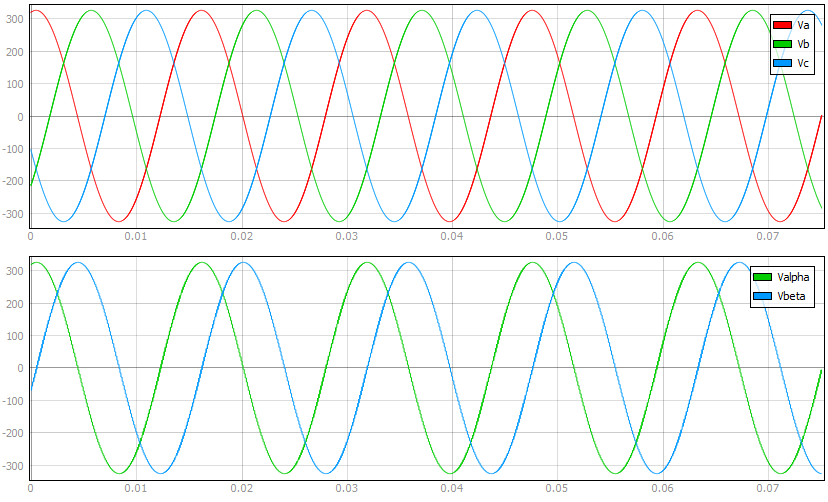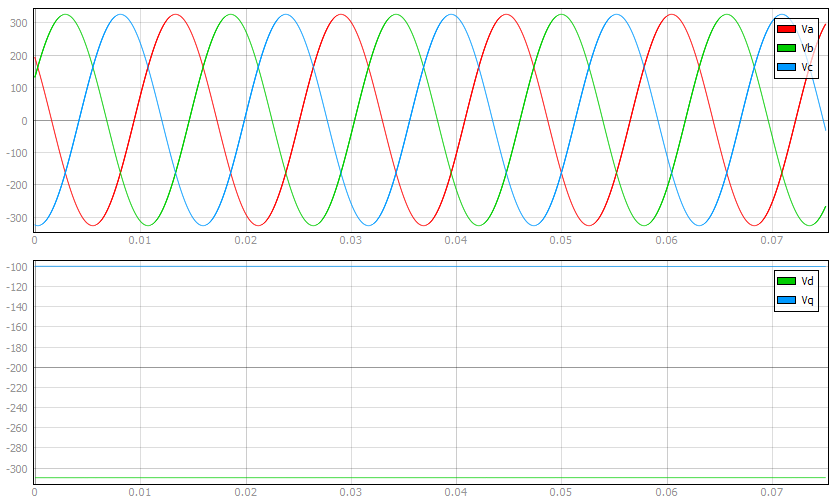# Machines

This section describes the concepts used in the modeling and implementation of electrical machine components in Typhoon HIL Schematic Editor. There are currently 26 different machine components.

All electrical machine components consist of two parts:
• the electrical circuit interface
• the machine solver
The signals measured in the circuit interface are used as inputs to the machine solver. The machine solver then calculates the model outputs and feeds them to the controlled sources in the circuit interface.

## Circuit interface

The machine components in the Typhoon HIL Schematic Editor Library use two types of circuit interfaces, the current source interface, and the voltage behind reactance interface (VBR). When using the current source interface, the machine model equations are formulated in such a way that the voltages are inputs, while the currents are outputs. Conversely, when using the voltage behind reactance interface, the machine model equations are formulated in such a way that the currents are inputs, while the voltages are outputs. Stator, rotor, and field winding variables can be found as machine_name.winding.variable_name in HIL SCADA (from the 2021.3 Typhoon HIL Control Center version).

Table 1. Current sources implementation variables
symbol description
v_ab Measured line voltage between terminals A and B [V]
v_bc Measured line voltage between terminals B and C [V]
i_ac Calculated phase A current [A]
i_bc Calculated phase B current [A]
Te Machine developed electromagnetic torque [Nm]
Table 2. Voltage behind reactance implementation variables
symbol description
i_a Measured phase A current [A]
i_b Measured phase B current [A]
i_c Measured phase C current [A]
e_ac Calculated phase A induced back emf [V]
e_bc Calculated phase B induced back emf [V]
e_cc Calculated phase C induced back emf [V]
Te Machine developed electromagnetic torque [Nm]

## Machine solver

The machine solver is a dedicated module that simulates the electrical and mechanical parts of the machine model, as well as the encoder and resolver feedback signals. Electrical subsystem model equations are used for calculating machine electrical variables. The motion equation and the machine electrical torque expression are used to calculate machine mechanical variables. Nonlinear effects such as flux saturation and spatial harmonics are modeled as lookup tables. The number of points available for the lookup tables used in a single machine component is 215. If the total number of points provided in the lookup tables exceeds this number, the tables will be rescaled to work with fewer points. The resulting tables may have a lower resolution than the original ones. The lookup tables use linear interpolation and linear extrapolation. More information about the nonlinear machines toolbox can be found here.

## Reference frames

Reference frame transformations are commonly used to eliminate time-varying inductances in the machine model. Unless otherwise noted, all of the machine models in the Typhoon HIL Schematic Editor Library are implemented using one of the following reference frames:

Stationary αβ reference frame

Rotating dq reference frame

## Stationary αβ reference frame

Clarke's transformation transforms stator and rotor electrical variables from the original three phase domain into a stationary two phase αβ domain.

Electrical variables are transformed to αβ components using the transformation matrix:

${T}_{\mathrm{abc}/\mathrm{\alpha \beta o}}=\frac{2}{3}\left[\begin{array}{ccc}cos{\theta }_{ab}& cos\left({\theta }_{ab}-\frac{2\pi }{3}\right)& cos\left({\theta }_{ab}+\frac{2\pi }{3}\right)\\ -sin{\theta }_{ab}& -sin\left({\theta }_{ab}-\frac{2\pi }{3}\right)& -sin\left({\theta }_{ab}+\frac{2\pi }{3}\right)\\ \frac{1}{2}& \frac{1}{2}& \frac{1}{2}\end{array}\right]$

In order to keep the same amplitude of the electrical variables as in the original phase domain, a multiplier of 2/3 is introduced in the abc/αβ transformation matrix. θab determines the position of the used stationary reference frame with regard to the stator phase winding a.

Three phase quantities are transformed to αβ components using the equation:

${X}_{\mathrm{\alpha \beta o}}={T}_{\mathrm{abc}/\mathrm{\alpha \beta o}}{٠X}_{\mathrm{abc}}$

where X represents a vector of voltages, currents, or fluxes.

Stationary αβ components of electrical variables are transformed to phase values using the inverse Clarke's transformation matrix in the following form:

${T}_{\mathrm{\alpha \beta o}/\mathrm{abc}}=\left[\begin{array}{ccc}cos{\theta }_{ab}& -sin{\theta }_{ab}& 1\\ cos\left({\theta }_{ab}-\frac{2\pi }{3}\right)& -sin\left({\theta }_{ab}-\frac{2\pi }{3}\right)& 1\\ cos\left({\theta }_{ab}+\frac{2\pi }{3}\right)& -sin\left({\theta }_{ab}+\frac{2\pi }{3}\right)& 1\end{array}\begin{array}{}\end{array}\begin{array}{c}\\ \\ \end{array}\right]$

αβ components of three phase quantities are transformed to phase components using the equation:

${X}_{\mathrm{abc}}={T}_{\mathrm{\alpha \beta o}/\mathrm{abc}}٠{X}_{\mathrm{\alpha \beta o}}$

where X represents a vector of voltages, currents, or fluxes.Figure 3. Transformation of a three phase frame into an αβ frame

The general form of the αβ transformation matrix for the n-phase machine is given by:

${T}_{\mathrm{abc}/\mathrm{\alpha \beta o}}=\frac{2}{n}\left[\begin{array}{cccccccc}cos{\theta }_{ab}& cos\left({\theta }_{ab}-\frac{2\pi }{n}\right)& cos\left({\theta }_{ab}-\frac{2٠2\pi }{n}\right)& cos\left({\theta }_{ab}-\frac{3٠2\pi }{n}\right)& ٠٠٠& cos\left({\theta }_{ab}-\frac{\left(n-3\right)٠2\pi }{n}\right)& cos\left({\theta }_{ab}-\frac{\left(n-2\right)٠2\pi }{n}\right)& cos\left({\theta }_{ab}-\frac{\left(n-1\right)٠2\pi }{n}\right)\\ -sin{\theta }_{ab}& -sin\left({\theta }_{ab}-\frac{2\pi }{n}\right)& -sin\left({\theta }_{ab}-\frac{2٠2\pi }{n}\right)& -sin\left({\theta }_{ab}-\frac{3٠2\pi }{n}\right)& ٠٠٠& -sin\left({\theta }_{ab}-\frac{\left(n-3\right)٠2\pi }{n}\right)& -sin\left({\theta }_{ab}-\frac{\left(n-2\right)٠2\pi }{n}\right)& -sin\left({\theta }_{ab}-\frac{\left(n-1\right)٠2\pi }{n}\right)\\ cos{\theta }_{ab}& cos\left({\theta }_{ab}-\frac{2٠2\pi }{n}\right)& cos\left({\theta }_{ab}-\frac{4٠2\pi }{n}\right)& cos\left({\theta }_{ab}-\frac{6٠2\pi }{n}\right)& ٠٠٠& cos\left({\theta }_{ab}-\frac{2٠\left(n-3\right)٠2\pi }{n}\right)& cos\left({\theta }_{ab}-\frac{2٠\left(n-2\right)٠2\pi }{n}\right)& cos\left({\theta }_{ab}-\frac{2٠\left(n-1\right)٠2\pi }{n}\right)\\ -sin{\theta }_{ab}& -sin\left({\theta }_{ab}-\frac{2٠2\pi }{n}\right)& -sin\left({\theta }_{ab}-\frac{4٠2\pi }{n}\right)& -sin\left({\theta }_{ab}-\frac{6٠2\pi }{n}\right)& ٠٠٠& -sin\left({\theta }_{ab}-\frac{2٠\left(n-3\right)٠2\pi }{n}\right)& -sin\left({\theta }_{ab}-\frac{2٠\left(n-2\right)٠2\pi }{n}\right)& -sin\left({\theta }_{ab}-\frac{2٠\left(n-1\right)٠2\pi }{n}\right)\\ cos{\theta }_{ab}& cos\left({\theta }_{ab}-\frac{3٠2\pi }{n}\right)& cos\left({\theta }_{ab}-\frac{6٠2\pi }{n}\right)& cos\left({\theta }_{ab}-\frac{9٠2\pi }{n}\right)& ٠٠٠& cos\left({\theta }_{ab}-\frac{3٠\left(n-3\right)٠2\pi }{n}\right)& cos\left({\theta }_{ab}-\frac{3٠\left(n-2\right)٠2\pi }{n}\right)& cos\left({\theta }_{ab}-\frac{3٠\left(n-1\right)٠2\pi }{n}\right)\\ -sin{\theta }_{ab}& -sin\left({\theta }_{ab}-\frac{3٠2\pi }{n}\right)& -sin\left({\theta }_{ab}-\frac{6٠2\pi }{n}\right)& -sin\left({\theta }_{ab}-\frac{9٠2\pi }{n}\right)& ٠٠٠& -sin\left({\theta }_{ab}-\frac{3٠\left(n-3\right)٠2\pi }{n}\right)& -sin\left({\theta }_{ab}-\frac{3٠\left(n-2\right)٠2\pi }{n}\right)& -sin\left({\theta }_{ab}-\frac{3٠\left(n-1\right)٠2\pi }{n}\right)\\ ٠٠٠& ٠٠٠& ٠٠٠& ٠٠٠& ٠٠٠& ٠٠٠& ٠٠٠& ٠٠٠\\ \frac{1}{2}& \frac{1}{2}& \frac{1}{2}& \frac{1}{2}& ٠٠٠& \frac{1}{2}& \frac{1}{2}& \frac{1}{2}\\ \frac{1}{2}& -\frac{1}{2}& \frac{1}{2}& -\frac{1}{2}& ٠٠٠& -\frac{1}{2}& \frac{1}{2}& -\frac{1}{2}\end{array}\right]$

where n is the number of phases.

The first two rows of the matrix define variables that will lead to fundamental flux and torque production. The last two rows define the two zero sequence components, and the last row of the transformation matrix is omitted for all odd phase numbers n. In between, there are (n - 4)/2 pairs of rows which define (n - 4)/2 pairs of variables for even phase numbers, and (n - 3)/2 pairs of rows and variables for odd phase numbers. These components correspond to certain voltage, flux, and current harmonics and do not contribute to torque production.

## Rotating dq reference frame

Park's transformation transforms stator and rotor electrical variables from the original three phase domain into a rotating two phase dq domain.

Electrical variables are transformed to dq components using the transformation matrix:

${T}_{\mathrm{abc}/\mathrm{dqo}}=\frac{2}{3}\left[\begin{array}{ccc}cos\left(\omega t+{\theta }_{ab}\right)& cos\left(\omega t-\frac{2\pi }{3}+{\theta }_{ab}\right)& cos\left(\omega t+\frac{2\pi }{3}+{\theta }_{ab}\right)\\ -sin\left(\omega t+{\theta }_{ab}\right)& -sin\left(\omega t-\frac{2\pi }{3}+{\theta }_{ab}\right)& -sin\left(\omega t+\frac{2\pi }{3}+{\theta }_{ab}\right)\\ \frac{1}{2}& \frac{1}{2}& \frac{1}{2}\end{array}\right]$

The position of the rotating frame is given by ωt, where ω represents the dq frame rotation speed. θab determines the position of the used rotating reference frame with regard to the stator phase winding a.

Three phase quantities are transformed to dq components using the equation:

${X}_{\mathrm{dqo}}={T}_{\mathrm{abc}/\mathrm{dqo}}٠{X}_{\mathrm{abc}}$

where X represents a vector of voltages, currents, or fluxes.

Rotating dq components of electrical variables are transformed to phase values using the inverse Park's transformation matrix in the following form:

${T}_{\mathrm{dqo}/\mathrm{abc}}=\left[\begin{array}{ccc}cos\left(\omega t+{\theta }_{ab}\right)& -sin\left(\omega t+{\theta }_{ab}\right)& 1\\ cos\left(\omega t-\frac{2\pi }{3}+{\theta }_{ab}\right)& -sin\left(\omega t-\frac{2\pi }{3}+{\theta }_{ab}\right)& 1\\ cos\left(\omega t+\frac{2\pi }{3}+{\theta }_{ab}\right)& -sin\left(\omega t+\frac{2\pi }{3}+{\theta }_{ab}\right)& 1\end{array}\right]$

dq components of three phase quantities are transformed to phase components using the equation:

${X}_{\mathrm{abc}}={T}_{\mathrm{dqo}/\mathrm{abc}}٠{X}_{\mathrm{dqo}}$

where X represents a vector of voltages, currents, or fluxes.Figure 4. Transformation of a three phase frame into dq frame

## List of Machine Library Components

The current version of Typhoon HIL Schematic Editor offers a selection of the following types of machine components:

Nine Phase Squirrel Cage Induction Machine

Single Phase Induction Machine

Six Phase Doubly Fed Induction Machine (Double Stator)

Three Phase Double Cage Induction Machine

Three Phase Doubly Fed Induction Machine

Three Phase Doubly Fed Induction Machine (VBR)

Three Phase Squirrel Cage Induction Machine

Three Phase Squirrel Cage Induction Machine (Open Winding)

Three Phase Squirrel Cage Induction Machine (SP)

Three Phase Squirrel Cage Induction Machine (VBR)

Nine Phase Permanent Magnet Synchronous Machine (Triple Stator)

Six Phase Permanent Magnet Synchronous Machine (Double Stator)

Six Phase Wound Rotor Synchronous Machine (Double Stator)

Three Phase Cylindrical Rotor Permanent Magnet Synchronous Machine

Three Phase Permanent Magnet Assisted Synchronous Reluctance Machine

Three Phase Permanent Magnet Synchronous Machine

Three Phase Permanent Magnet Synchronous Machine (JMAG)

Three Phase Permanent Magnet Synchronous Machine (Open Winding)

Three Phase Permanent Magnet Synchronous Machine (VBR)

Three Phase Permanent Magnet Synchronous Machine with Neutral Connection

Three Phase Wound Rotor Synchronous Machine

Three Phase Wound Rotor Synchronous Machine (Generic)

Three Phase Wound Rotor Synchronous Machine (Open Winding)

Three Phase Wound Rotor Synchronous Machine with Neutral Connection

Three Phase Wound Rotor Synchronous Machine with Neutral Connection (VBR)

Brushless DC Machine

Separate or Parallel Excitation DC Machine

Permanent Magnet DC Machine

Hall Effect Sensor

Three Phase Stepper Motor

Three Phase Switched Reluctance Machine

## References

Machine components were implemented based on the principles described in the following literature:

• Paul C. Krause., Oleg Wasynczuk, and Scott D. Sudhoff, Analysis of Electric Machinery, IEEE Press, 2002.
• Liwei Wang, Modeling of AC machines using a voltage-behind-reactance formulation of electromagnetic transients in power systems, The University of British Columbia, Vancouver, 2010.
• Robert Vartanian, Permanent Magnet Assisted Synchronous Reluctance Machine (PMa-SynRM) Design and Performance Analysis for Fan and Pump Applications, Doctoral dissertation at Texas A & M University, 2014.
• Xiao Chen, Jiabin Wang, Bhaskar Sen, Panagiotis Lazari, Tianfu Sun, A High-Fidelity and Computationally Efficient Model for Interior Permanent-Magnet Machines Considering the Magnetic Saturation, Spatial Harmonics, and Iron Loss Effect, IEEE, 2015.
• I. M. Canay, Causes of Discrepancies on Calculation of Rotor Quantities and Exact Equivalent Diagrams of the Synchronous Machine, IEEE, 1969.
• Alberto Berzoy, Ahmed A. S. Mohamed, Osama Mohammed, Complex-Vector Model of Interturn Failure in Induction Machines for Fault Detection and Identification, IEEE, 2016.
• Liwei Wang, Juri Jatskevich, Steven D. Pekarek, Modeling of Induction Machines Using a Voltage-Behind-Reactance Formulation, IEEE, 2008.
• Emil Levi, Radu Bojoi, F. Profumo, Hamid Toliyat, Multiphase induction motor drives - A technology status review, IET Electric Power Applications, 2007.